# PSAT Math : How to use the inverse variation formula

## Example Questions

### Example Question #1 : How To Use The Inverse Variation Formula

The square ofvaries inversely with the cube of. If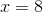when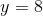, then what is the value ofwhen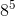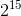Explanation:

When two quantities vary inversely, their products are always equal to a constant, which we can call k. If the square of x and the cube of y vary inversely, this means that the product of the square of x and the cube of y will equal k. We can represent the square of x as x2 and the cube of y as y3. Now, we can write the equation for inverse variation.

x2y3 = k

We are told that when x = 8, y = 8. We can substitute these values into our equation for inverse variation and then solve for k.

82(83) = k

k = 82(83)

Because this will probably be a large number, it might help just to keep it in exponent form. Let's apply the property of exponents which says that abac = ab+c.

k = 82(83) = 82+3 = 85.

Next, we must find the value of y when x = 1. Let's use our equation for inverse variation equation, substituting 85 in for k.

x2y3 = 85

(1)2y3 = 85

y3 = 85

In order to solve this, we will have to take a cube root. Thus, it will help to rewrite 8 as the cube of 2, or 23.

y3 = (23)5

We can now apply the property of exponents that states that (ab)c = abc.

y3 = 23•5 = 215

In order to get y by itself, we will have the raise each side of the equation to the 1/3 power.

(y3)(1/3) = (215)(1/3)

Once again, let's apply the property (ab)c = abc.

y(3 • 1/3) = 2(15 • 1/3)

y = 25 = 32

### Example Question #1 : How To Use The Inverse Variation Formulavaries inversely as both the square ofand the square root of. Assuming thatdoes not depend on any other variable, which statement is true ofconcerning its relationship to?varies inversely as the fourth root of.varies inversely as.varies directly as the fourth root of.varies inversely as the fourth power of.varies directly as the fourth power of.varies inversely as the fourth power of.

Explanation:varies inversely as both the square ofand the square root of, meaning that for some constant of variation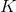,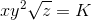.

Square both sides, and the expression becomes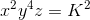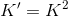takes the role of the new constant of variation here, and we now have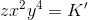,

meaning thatvaries inversely as the fourth power of.

### Example Question #1 : Direct And Inverse Variation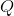varies directly as the square ofand inversely as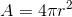and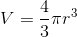. Assuming thatdoes not depend on any other variables, which of the following gives the variation relationship ofto?varies inversely as.varies directly as the fourth power of.varies inversely as the seventh power of.varies inversely as the fourth power of.varies directly as.varies directly as.

Explanation:varies directly as the square ofand inversely as; therefore, for some constant of variation,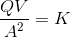Settingand, the formula becomes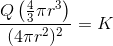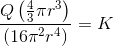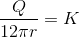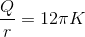Setting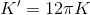as the new constant of variation, we have a new variation equation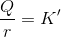,

meaning thatvaries directly as.